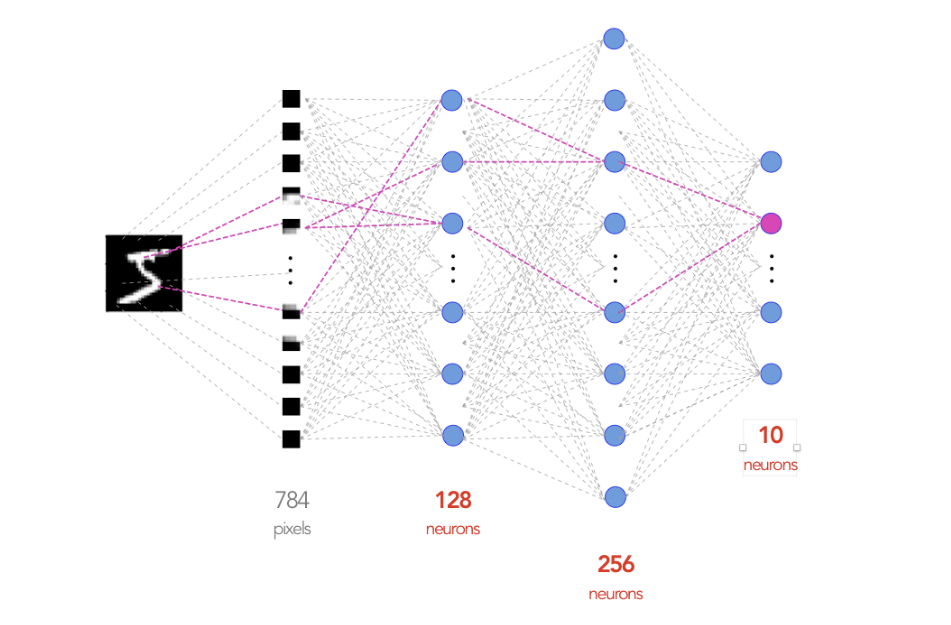# From PyTorch to PyTorch Lightning — A gentle introduction# The Typical AI Research project

In a research project, we normally want to identify the following key components:

• the model(s)
• the data
• the loss
• the optimizer(s)

# The Model

Let’s design a 3-layer fully-connected neural network that takes as input an image that is 28×28 and outputs a probability distribution over 10 possible labels.

First, let’s define the model in PyTorch